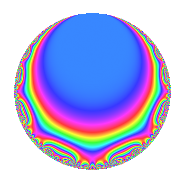# Properties

 Label 4012.2.a.fLevel 4012 Weight 2 Character orbit 4012.a Self dual Yes Analytic conductor 32.036 Analytic rank 1 Dimension 3 CM No Inner twists 1

# Related objects

## Newspace parameters

 Level: $$N$$ = $$4012 = 2^{2} \cdot 17 \cdot 59$$ Weight: $$k$$ = $$2$$ Character orbit: $$[\chi]$$ = 4012.a (trivial)

## Newform invariants

 Self dual: Yes Analytic conductor: $$32.0359812909$$ Analytic rank: $$1$$ Dimension: $$3$$ Coefficient field: 3.3.321.1 Coefficient ring: $$\Z[a_1, \ldots, a_{23}]$$ Coefficient ring index: $$2^{2}$$ Fricke sign: $$1$$ Sato-Tate group: $\mathrm{SU}(2)$

## $q$-expansion

Coefficients of the $$q$$-expansion are expressed in terms of a basis $$1,\beta_1,\beta_2$$ for the coefficient ring described below. We also show the integral $$q$$-expansion of the trace form.

 $$f(q)$$ $$=$$ $$q$$ $$- q^{3}$$ $$-\beta_{1} q^{5}$$ $$+ q^{7}$$ $$-2 q^{9}$$ $$+O(q^{10})$$ $$q$$ $$- q^{3}$$ $$-\beta_{1} q^{5}$$ $$+ q^{7}$$ $$-2 q^{9}$$ $$-2 q^{11}$$ $$+ ( 1 + \beta_{1} ) q^{13}$$ $$+ \beta_{1} q^{15}$$ $$+ q^{17}$$ $$+ ( -2 + \beta_{1} ) q^{19}$$ $$- q^{21}$$ $$+ ( -\beta_{1} - \beta_{2} ) q^{23}$$ $$+ ( 6 + 2 \beta_{2} ) q^{25}$$ $$+ 5 q^{27}$$ $$+ ( -2 - \beta_{1} ) q^{29}$$ $$+ ( 2 + \beta_{1} - \beta_{2} ) q^{31}$$ $$+ 2 q^{33}$$ $$-\beta_{1} q^{35}$$ $$+ ( 3 + 2 \beta_{1} - \beta_{2} ) q^{37}$$ $$+ ( -1 - \beta_{1} ) q^{39}$$ $$+ ( -2 + \beta_{1} ) q^{41}$$ $$+ ( -2 + \beta_{1} - \beta_{2} ) q^{43}$$ $$+ 2 \beta_{1} q^{45}$$ $$+ ( -4 + \beta_{1} + \beta_{2} ) q^{47}$$ $$-6 q^{49}$$ $$- q^{51}$$ $$+ ( 5 + 2 \beta_{2} ) q^{53}$$ $$+ 2 \beta_{1} q^{55}$$ $$+ ( 2 - \beta_{1} ) q^{57}$$ $$- q^{59}$$ $$+ ( -3 - \beta_{1} ) q^{61}$$ $$-2 q^{63}$$ $$+ ( -11 - \beta_{1} - 2 \beta_{2} ) q^{65}$$ $$+ ( -2 + 2 \beta_{1} + 2 \beta_{2} ) q^{67}$$ $$+ ( \beta_{1} + \beta_{2} ) q^{69}$$ $$+ ( -2 + 2 \beta_{2} ) q^{71}$$ $$+ 2 q^{73}$$ $$+ ( -6 - 2 \beta_{2} ) q^{75}$$ $$-2 q^{77}$$ $$-5 q^{79}$$ $$+ q^{81}$$ $$+ ( -1 + 2 \beta_{1} - \beta_{2} ) q^{83}$$ $$-\beta_{1} q^{85}$$ $$+ ( 2 + \beta_{1} ) q^{87}$$ $$+ ( 4 + \beta_{1} - \beta_{2} ) q^{89}$$ $$+ ( 1 + \beta_{1} ) q^{91}$$ $$+ ( -2 - \beta_{1} + \beta_{2} ) q^{93}$$ $$+ ( -11 + 2 \beta_{1} - 2 \beta_{2} ) q^{95}$$ $$+ ( -3 + \beta_{1} - 2 \beta_{2} ) q^{97}$$ $$+ 4 q^{99}$$ $$+O(q^{100})$$ $$\operatorname{Tr}(f)(q)$$ $$=$$ $$3q$$ $$\mathstrut -\mathstrut 3q^{3}$$ $$\mathstrut +\mathstrut q^{5}$$ $$\mathstrut +\mathstrut 3q^{7}$$ $$\mathstrut -\mathstrut 6q^{9}$$ $$\mathstrut +\mathstrut O(q^{10})$$ $$3q$$ $$\mathstrut -\mathstrut 3q^{3}$$ $$\mathstrut +\mathstrut q^{5}$$ $$\mathstrut +\mathstrut 3q^{7}$$ $$\mathstrut -\mathstrut 6q^{9}$$ $$\mathstrut -\mathstrut 6q^{11}$$ $$\mathstrut +\mathstrut 2q^{13}$$ $$\mathstrut -\mathstrut q^{15}$$ $$\mathstrut +\mathstrut 3q^{17}$$ $$\mathstrut -\mathstrut 7q^{19}$$ $$\mathstrut -\mathstrut 3q^{21}$$ $$\mathstrut +\mathstrut 20q^{25}$$ $$\mathstrut +\mathstrut 15q^{27}$$ $$\mathstrut -\mathstrut 5q^{29}$$ $$\mathstrut +\mathstrut 4q^{31}$$ $$\mathstrut +\mathstrut 6q^{33}$$ $$\mathstrut +\mathstrut q^{35}$$ $$\mathstrut +\mathstrut 6q^{37}$$ $$\mathstrut -\mathstrut 2q^{39}$$ $$\mathstrut -\mathstrut 7q^{41}$$ $$\mathstrut -\mathstrut 8q^{43}$$ $$\mathstrut -\mathstrut 2q^{45}$$ $$\mathstrut -\mathstrut 12q^{47}$$ $$\mathstrut -\mathstrut 18q^{49}$$ $$\mathstrut -\mathstrut 3q^{51}$$ $$\mathstrut +\mathstrut 17q^{53}$$ $$\mathstrut -\mathstrut 2q^{55}$$ $$\mathstrut +\mathstrut 7q^{57}$$ $$\mathstrut -\mathstrut 3q^{59}$$ $$\mathstrut -\mathstrut 8q^{61}$$ $$\mathstrut -\mathstrut 6q^{63}$$ $$\mathstrut -\mathstrut 34q^{65}$$ $$\mathstrut -\mathstrut 6q^{67}$$ $$\mathstrut -\mathstrut 4q^{71}$$ $$\mathstrut +\mathstrut 6q^{73}$$ $$\mathstrut -\mathstrut 20q^{75}$$ $$\mathstrut -\mathstrut 6q^{77}$$ $$\mathstrut -\mathstrut 15q^{79}$$ $$\mathstrut +\mathstrut 3q^{81}$$ $$\mathstrut -\mathstrut 6q^{83}$$ $$\mathstrut +\mathstrut q^{85}$$ $$\mathstrut +\mathstrut 5q^{87}$$ $$\mathstrut +\mathstrut 10q^{89}$$ $$\mathstrut +\mathstrut 2q^{91}$$ $$\mathstrut -\mathstrut 4q^{93}$$ $$\mathstrut -\mathstrut 37q^{95}$$ $$\mathstrut -\mathstrut 12q^{97}$$ $$\mathstrut +\mathstrut 12q^{99}$$ $$\mathstrut +\mathstrut O(q^{100})$$

Basis of coefficient ring in terms of a root $$\nu$$ of $$x^{3}\mathstrut -\mathstrut$$ $$x^{2}\mathstrut -\mathstrut$$ $$4$$ $$x\mathstrut +\mathstrut$$ $$1$$:

 $$\beta_{0}$$ $$=$$ $$1$$ $$\beta_{1}$$ $$=$$ $$2 \nu - 1$$ $$\beta_{2}$$ $$=$$ $$2 \nu^{2} - 2 \nu - 5$$
 $$1$$ $$=$$ $$\beta_0$$ $$\nu$$ $$=$$ $$($$$$\beta_{1}\mathstrut +\mathstrut$$ $$1$$$$)/2$$ $$\nu^{2}$$ $$=$$ $$($$$$\beta_{2}\mathstrut +\mathstrut$$ $$\beta_{1}\mathstrut +\mathstrut$$ $$6$$$$)/2$$

## Embeddings

For each embedding $$\iota_m$$ of the coefficient field, the values $$\iota_m(a_n)$$ are shown below.

For more information on an embedded modular form you can click on its label.

Label $$\iota_m(\nu)$$ $$a_{2}$$ $$a_{3}$$ $$a_{4}$$ $$a_{5}$$ $$a_{6}$$ $$a_{7}$$ $$a_{8}$$ $$a_{9}$$ $$a_{10}$$
1.1
 2.46050 0.239123 −1.69963
0 −1.00000 0 −3.92101 0 1.00000 0 −2.00000 0
1.2 0 −1.00000 0 0.521753 0 1.00000 0 −2.00000 0
1.3 0 −1.00000 0 4.39926 0 1.00000 0 −2.00000 0
 $$n$$: e.g. 2-40 or 990-1000 Significant digits: Format: Complex embeddings Normalized embeddings Satake parameters Satake angles

## Inner twists

This newform does not admit any (nontrivial) inner twists.

## Atkin-Lehner signs

$$p$$ Sign
$$2$$ $$-1$$
$$17$$ $$-1$$
$$59$$ $$1$$

## Hecke kernels

This newform can be constructed as the intersection of the kernels of the following linear operators acting on $$S_{2}^{\mathrm{new}}(\Gamma_0(4012))$$:

 $$T_{3}$$ $$\mathstrut +\mathstrut 1$$ $$T_{5}^{3}$$ $$\mathstrut -\mathstrut T_{5}^{2}$$ $$\mathstrut -\mathstrut 17 T_{5}$$ $$\mathstrut +\mathstrut 9$$